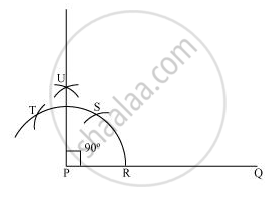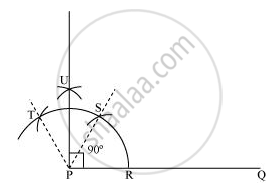Share

# Construct an Angle of 90° at the Initial Point of a Given Ray and Justify the Construction. - CBSE Class 9 - Mathematics

ConceptBasic Constructions

#### Question

Construct an angle of 90° at the initial point of a given ray and justify the construction.

#### Solution

The below given steps will be followed to construct an angle of 90°.

(i) Take the given ray PQ. Draw an arc of some radius taking point P as its centre, which intersects PQ at R.

(ii) Taking R as centre and with the same radius as before, draw an arc intersecting the previously drawn arc at S.

(iii) Taking S as centre and with the same radius as before, draw an arc intersecting the arc at T (see figure).

(iv) Taking S and T as centre, draw an arc of same radius to intersect each other at U.

(v) Join PU, which is the required ray making 90° with the given ray PQ.Justification of Construction:

We can justify the construction, if we can prove ∠UPQ = 90°.

For this, join PS and PT.We have, ∠SPQ = ∠TPS = 60°. In (iii) and (iv) steps of this construction, PU was drawn as the bisector of ∠TPS.

∴ ∠UPS = 1/2 ∠TPS = 1/2*60° = 30°

Also, ∠UPQ = ∠SPQ + ∠UPS

= 60° + 30°

= 90°

Is there an error in this question or solution?

#### APPEARS IN

NCERT Solution for Mathematics Class 9 (2018 to Current)
Chapter 11: Constructions
Ex. 11.10 | Q: 1 | Page no. 191

#### Video TutorialsVIEW ALL 

Solution Construct an Angle of 90° at the Initial Point of a Given Ray and Justify the Construction. Concept: Basic Constructions.
S